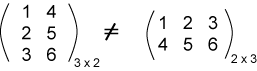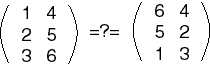No, to be equal, two matrices must have the same dimensions, and must have the same values in the same positions.

# Matrix Equality

For two matrices to be equal, they must have

1. The same dimensions.
2. Corresponding elements must be equal.

In other words, say that An x m = [aij] and that Bp x q = [bij].

Then A = B if and only if n=p, m=q, and aij=bij for all i and j in range.

Here are two matrices which are not equal even though they have the same elements.### QUESTION 6:

Here is another pair of matrices.Are these two matrices equal?